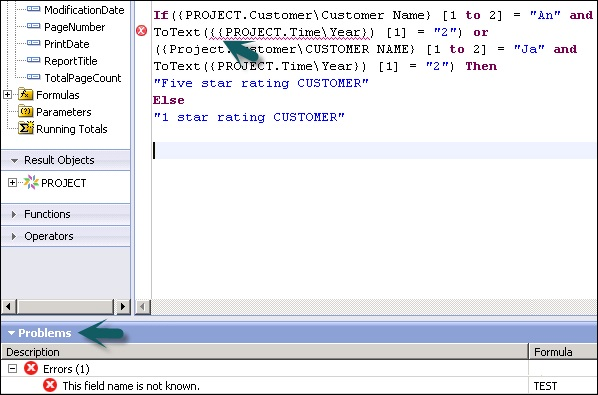# Creating & Modifying Formulas

You can create single or multiple formulas in one go and use them in your report. You can also delete the formulas, or search and change text of formulas in the formula text window.

To create a new formula, you can right click on Formula tab under Data Explorer. Once you click on the new formula → enter the formula name → it opens the formula workshop.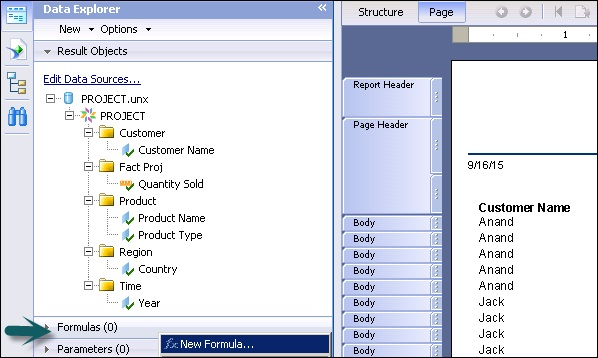You can also create a new formula by opening the Formula workshop from the Formula tab under Data → New → New Formula as shown in the following image.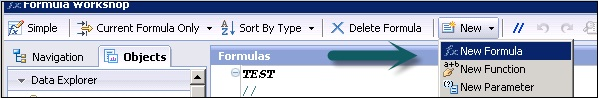To write a formula in the formula text window, you can use in-use objects under the Operator tab and different operators to pass condition in the formula.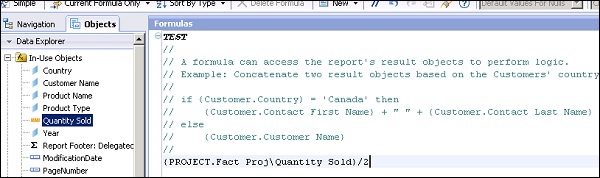A formula can be saved using the save option at the bottom of the page. Once the formula is saved it comes under the list of formulas in Data Explorer tab.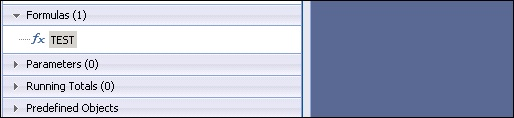You can drag this formula to any section of the report.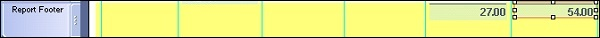In the above snapshot, Test formula has been dragged to Report footer and it has calculated value as Quantity sold/2 in the Report footer.

Now to modify the formula, click on formula name under Data explorer, it will open the Formula workshop. Make changes to formula and use the save button at the bottom to save the changes. These changes will be automatically applied to the Report values.

To delete the formula from a report, right click on the formula name and click on Delete.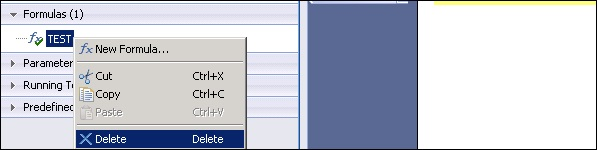Problem pane at bottom is used for debugging purpose. It shows you the syntax error in the formula. The error message in the following image says that the field in red underline is not known as field syntax and is incorrect.